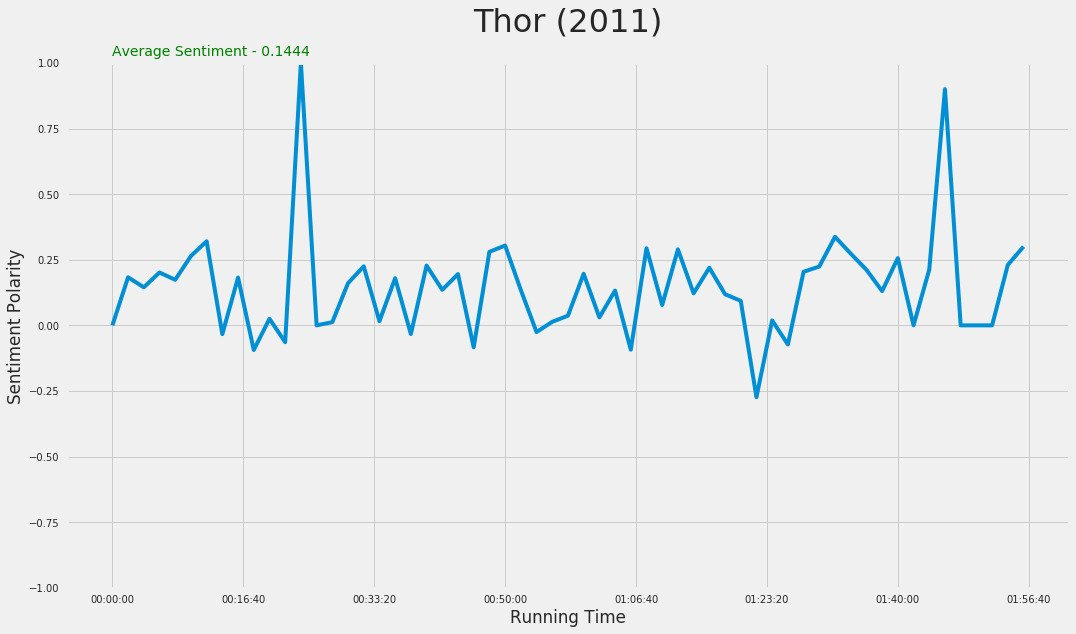# Analyzing Movie Subtitles

04 November 2017 — Written by Mubaris NK

In this post we will analyze a movie using sentiment analysis of its subtitles. This is little bit tricky. Because, the subtitles are time dependant data. Unlike other time series data, subtitles don't have constant time intervals. We will need to change them to equal time intervals. We will use various Python modules to analyze the subtitles. Let's start with importing the subtitle.

## pysrt - Python Module for Handling srt Subtitles

pysrt is a great Python module to handle srt files. Let's see pysrt in action.

import pysrt

subs = pysrt.open('some/file.srt')

sub = subs

# Subtitle text
text = sub.text
text_without_tags = sub.text_without_tags

# Start and End time
start = sub.start.to_time()
end = sub.end.to_time()

# Removing line and saving
del subs[index]
subs.save('some/file.srt')

## TextBlob - Simplified Python Module for Text Processing

TextBlob is a Python library for processing textual data. It provides a simple API for diving into common natural language processing (NLP) tasks such as part-of-speech tagging, noun phrase extraction, sentiment analysis, classification, translation, and more. Let's see TextBlob in action.

from textblob import TextBlob

text = '''
The titular threat of The Blob has always struck me as the ultimate movie
monster: an insatiably hungry, amoeba-like mass able to penetrate
virtually any safeguard, capable of--as a doomed doctor chillingly
describes it--"assimilating flesh on contact.
Snide comparisons to gelatin be damned, it's a concept with the most
devastating of potential consequences, not unlike the grey goo scenario
proposed by technological theorists fearful of
artificial intelligence run rampant.
'''

blob = TextBlob(text)
blob.tags           # [('The', 'DT'), ('titular', 'JJ'),
#  ('threat', 'NN'), ('of', 'IN'), ...]

blob.noun_phrases   # WordList(['titular threat', 'blob',
#            'ultimate movie monster',
#            'amoeba-like mass', ...])

for sentence in blob.sentences:
print(sentence.sentiment.polarity)
# 0.060
# -0.341

blob.translate(to="es")  # 'La amenaza titular de The Blob...'

# Sentiment Analysis
blob = TextBlob(text)
sentiment_polarity = blob.sentiment.polarity # -0.1590909090909091
sentiment_subjectivity = blob.sentiment.subjectivity # 0.6931818181818182

## The Method

We can divide the method in to 4 steps.

• Divide total running time to constant time intervals
• Collect and combine all the text in each time interval
• Find the sentiment polarity of text in each time interval
• Visualize our analysis
from datetime import date, datetime, timedelta, time
import pysrt
from textblob import TextBlob
import matplotlib
from matplotlib import style
import matplotlib.pyplot as plt
import seaborn as sns
sns.set()
%matplotlib inline
matplotlib.rcParams['figure.figsize'] = (16.0, 9.0)
style.use('fivethirtyeight')
# Helper Function to create equally divided time intervals
# start - Starting Time
# end - Ending Time
# delta - Interval Period
def create_intervals(start, end, delta):
curr = start
while curr <= end:
curr = (datetime.combine(date.today(), curr) + delta).time()
yield curr

# Main Function to Get Sentiment Data
# file - srt file location
# delta - time interval in minutes
def get_sentiment(file, delta=2):
subs = pysrt.open(file, encoding='iso-8859-1')
n = len(subs)
# List to store the time periods
intervals = []
# Start, End and Delta
start = time(0, 0, 0)
end = subs[-1].end.to_time()
delta = timedelta(minutes=delta)
for result in create_intervals(start, end, delta):
intervals.append(result)
# List to store sentiment polarity
sentiments = []

index = 0
m = len(intervals)
# Collect and combine all the text in each time interval
for i in range(m):
text = ""
for j in range(index, n):
# Finding all subtitle text in the each time interval
if subs[j].end.to_time() < intervals[i]:
text += subs[j].text_without_tags + " "
else:
break
# Sentiment Analysis
blob = TextBlob(text)
pol = blob.sentiment.polarity
sentiments.append(pol)
index = j
intervals.insert(0, time(0, 0, 0))
sentiments.insert(0, 0.0)
return (intervals, sentiments)

# Utility to find average sentiment
def average(y):
avg = float(sum(y))/len(y)
return avg

We have written our function to find sentiment of subtitle over interval of time. Now let's try to plot this. We'll use "Thor" movie subtitles here.

x, y = get_sentiment("Thor.srt")
fig, ax = plt.subplots()
plt.plot(x, y)
plt.title("Thor (2011)", fontsize=32)
plt.ylim((-1, 1))
plt.ylabel("Sentiment Polarity")
plt.xlabel("Running Time")
plt.text(.5, 1.03, "Average Sentiment - " + str(round(average(y), 4)), color="green")
ttl = ax.title
ttl.set_position([.5, 1.05])There we have our plot of Sentiment Analysis of subtitle against the running time. All code is available on GitHub

## More Examples

#### Iron Man (2008)#### The Incredible Hulk (2008)#### Iron Man 2 (2010)#### Thor (2011)#### Captain America: The First Avenger (2011)#### The Avengers (2012)#### Iron Man 3 (2013)#### Captain America: The Winter Soldier (2014)#### Guardians of the Galaxy (2014)#### Avengers: Age of Ultron (2015)#### Ant-Man (2015)#### Captain America: Civil War (2016)#### Doctor Strange (2016)#### Guardians of the Galaxy Vol. 2 (2017)#### Spider-Man: Homecoming (2017)#### Man of Steel (2013)#### Batman v Superman: Dawn of Justice (2016)